# 动态规划的引入

​ ——百度百科

• 阶段 问题被划分为若干阶段，每阶段受先前阶段影响
• 状态 描述该阶段特定子问题的若干变量
• 决策 从一个状态演变到下一阶段某个状态的选择
• 最优子结构 一个最优化策略的子策略总是最优的
• 无后效性 已经求解的子问题，不会再受到后续决策的影响

OI 中 DP 的含义被极大地扩展了，下面提取两大核心特征

• 状态 一个含义清晰且独立 (即无后效性) 的子问题 $I$

▶ 使用 $$f_I$$ 表示子问题 $I$ 的答案

▶ 可能需要引入辅助子问题 $g_J, h_K, · · ·$

• 转移 答案 $$f_I$$ 通过状态转移方程由其它子问题共同计算得到

▶ 以状态为点，转移为边，构成有向无环图 (DAG)

▶ 边界条件与平凡子问题

▶ 解空间中的任意元素都被恰当考虑

• 最优化 (k-优) 最优解能被考虑到，这依赖于最优子结构
• 计数 (概率, 期望) 计数对象 (事件) 能被不重不漏地处理

1.最优子结构性质。动态规划下一阶段的最优解应该能由前面已经算出的各阶段的最优解导出。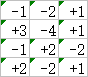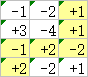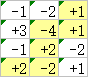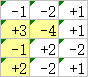2.无后效性。动态规划要求已经求解出的子问题不能受后续阶段的影响，也就是说，动态规划时对于状态空间的遍历应该构成一个有向无环图。3.子问题重叠。动态规划之所以优于爆搜，就是因为它以空间换时间的形式记录了前面所有状态的最优解。

#### Number Triangles

##### 题目描述

     7
3   8
8   1   0
2   7   4   4
4   5   2   6   5


##### 样例 #1
###### 样例输入 #1
5
7
3 8
8 1 0
2 7 4 4
4 5 2 6 5

###### 样例输出 #1
30


【数据范围】 对于 $$100\%$$ 的数据，$$1\le r \le 1000$$，所有输入在 $$[0,100]$$ 范围内。

int solve(int i, int j) {
return a[i][j] + (i == n ? 0 : max(solve(i + 1, j), solve(i + 1, j + 1)));
}


#include<bits/stdc++.h>
using namespace std;
int a;
int main() {
int n = 0;
cin >> n;
for (int i = 0; i < n; i++)
for (int j = 0; j <= i; j++)
cin >> f[i][j];
for (int i = n - 2; i >= 0; i--)
for (int j = 0; j <= i; j++)
f[i][j] += max(f[i + 1][j], f[i + 1][j + 1]);
cout << f;
}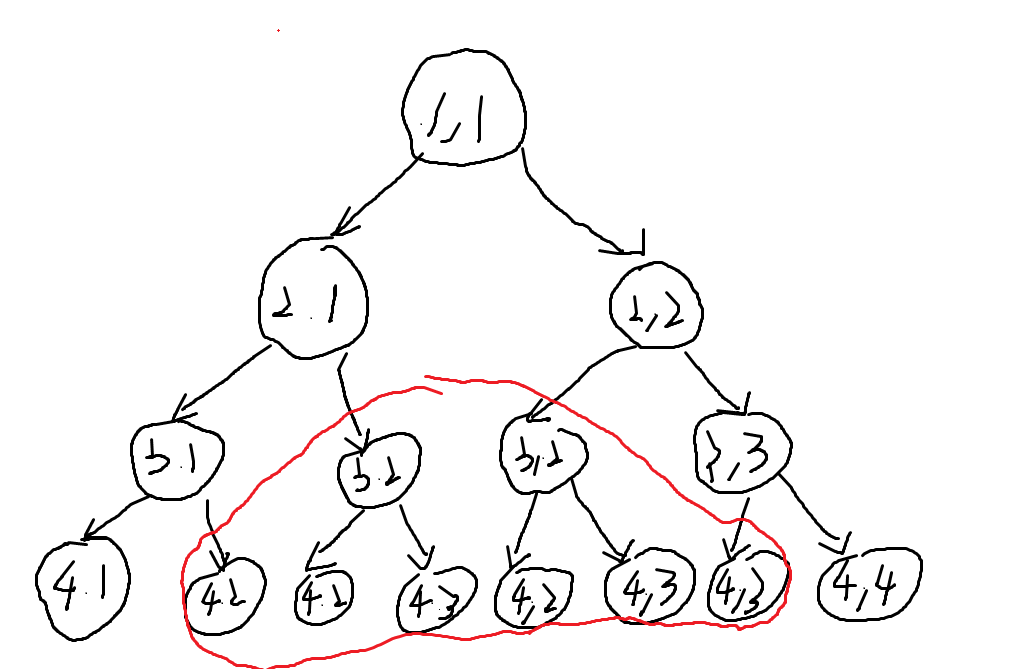memset(f, -1, sizeof(f));
int solve(int i, int j) {
if (f[i][j] >= 0) return f[i][j];
return f[i][j] = a[i][j] + (i == n ? 0 :
max(solve(i + 1, j), solve(i + 1, j + 1)));
}